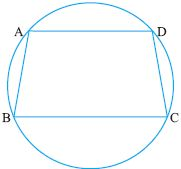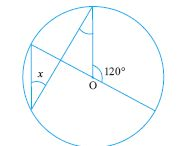Name: ___________________Date:___________________

 Email us to get an instant 20% discount on highly effective K-12 Math & English kwizNET Programs!

High School Mathematics - 28.6 Circles - Theorems

 Statement: If two arcs are congruent, then their chords are equal. Given: Arc ASB = Arc CTD To prove: AB = CDProof: OA = OlC, OB = OlD (radii) Angle AOB = Angle COlD (given that the arcs are congruent) Hence by SAS Postulate on congruence AOB is congruent to COlD Hence AB = CD Statement: There is only one circle passing through three non collinear points.Example: In the given figure prove that AB = CDSolution: Arc BAD congruent Arc CDA Hence Arc BAD - Arc AD = Arc CAD - Arc AD Arc BA is congruent Arc CD Hence AB = CD Directions: Solve the following.
 Q 1: BC is a chord of circle with centre O. A is a point on the major arc BC as shown, prove that angBAC + angOBC = 90.Answer: Q 2: BC is a chord of circle with centre O. A is a point on the minor arc BC as shown, prove that angBAC - angOBC = 90.Answer: Q 3: Two circles intersect each other at points A and B, if AP and AQ be the respective diameters of the circle prove that PBQ is a line.Answer: Q 4: Two congruent circles intersect each other at points P and Q, a line through P meets the circles in A and B. Prove that QA = QBAnswer: Q 5: AC and BD are chords of a circle that bisect each other. Prove that AC and BD are diameters.Answer: Q 6: Find angle x35o90o55o Q 7: Find the missing angle.35o30o55o Q 8: In a circle containing the equal chords AB and CD which are on the opposite side of the centre, E. Prove that BE = DE and AE = CE where E is the point of intersection of AD and BC.Answer: Question 9: This question is available to subscribers only! Question 10: This question is available to subscribers only!